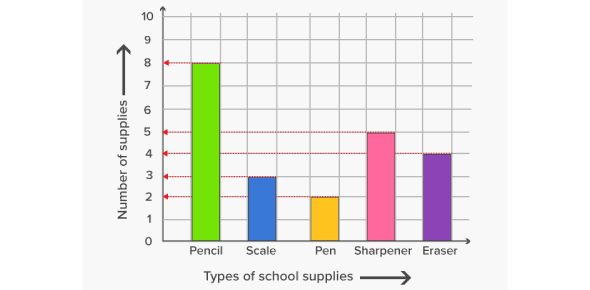# Graph Quiz! Trivia Knowledge Test! Questions

15 Questions | Total Attempts: 3640SettingsHow knowledgeable are you about graphs? This quiz will reveal your graph IQ. In mathematics, and more explicitly in graph theory, a graph is a structure that amounts to a set of objects that are, in some sense, “related. ” The objects correspond to mathematical abstractions known as vertices, and each of the related pairs of vertices is called an edge. If you understand this complex subject, then you will benefit from taking this quiz.

• 1.
What is the purpose of a line graph?
• A.

To show changes over periods of time

• B.

Shows comparison in relation to other data sets

• C.

Used to plot frequencies of occurrence in a continuous data set

• D.

Used to display and compare numerical data

• 2.
What type of graph is this?
• A.

Bar graph

• B.

Scatter plot

• C.

Line graph

• D.

Histogram

• 3.
What are the components of a pie chart?
• A.

Title

• B.

Scales

• C.

Key

• D.

Bars

• E.

Line

• F.

Circle

• G.

Points

• H.

Line of best fit

• 4.
What is the purpose of a scatter plot?
• A.

Clearly display proportions of data

• B.

Shows trends, relationships and results

• C.

Shows information given at a point in time

• D.

Helps visualize density of individual data values around particular points

• 5.
What are the components of a bar graph?
• A.

Title

• B.

Line of best fit

• C.

Intervals

• D.

Labels

• E.

Bars

• F.

Key

• G.

Colors

• H.

Line

• 6.
What is the purpose of a histogram?
• A.

To compare individual data to the whole

• B.

Graphically summarizes distribution through grouping

• C.

Helps visualize density of individual data values around particular points

• D.

Visual comparison of two variables

• 7.
What are the components of a bar graph/histogram?
• A.

Title

• B.

Bars

• C.

Colors

• D.

Points

• E.

Labels

• F.

Intervals

• 8.
What are the components of a scatter plot?
• A.

Title

• B.

Labels

• C.

Intervals

• D.

Points

• E.

Line of best fit

• F.

Box

• G.

Colors

• H.

Bars

• 9.
What is the purpose of a pie chart?
• A.

Shows comparison in relation to other data sets

• B.

Visual comparison of two variables

• C.

Used to plot frequencies of occurrence in a continuous data set

• D.

Helps visualize density of individual data values around particular points

• 10.
Which type of graph represents size relationship between parts and the whole?
• A.

Bar graph

• B.

Scatter plot

• C.

Pie chart

• D.

Line graph

• 11.
What is this type of graph?
• A.

Line graph

• B.

Bar graph

• C.

Pie chart

• D.

Histogram

• 12.
What are two things all graphs require?
• A.

Title

• B.

Bars

• C.

Data

• D.

Line

• E.

Scales

• F.

Key

• 13.
What is the purpose of a bar graph?
• A.

Represents size relationship between parts and the whole of the data

• B.

Shows how one variable is connected to another

• C.

Visual comparison of two variables

• D.

Used to display and compare numerical data

• 14.
What is this type of graph?
• A.

Bar graph

• B.

Scatter plot

• C.

Line graph

• D.

Pie chart

• 15.
What is this type of graph?
• A.

Histogram

• B.

Pie chart

• C.

Line graph

• D.

Scatter plot

Related TopicsBack to top